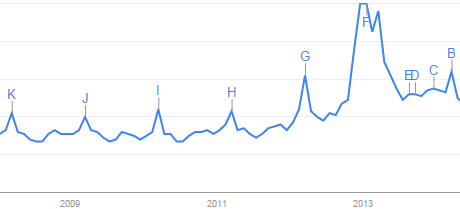Search IntMath
Close

450+ Math Lessons written by Math Professors and Teachers

5 Million+ Students Helped Each Year

1200+ Articles Written by Math Educators and Enthusiasts

Simplifying and Teaching Math for Over 23 Years

# A peak of pi

By Murray Bourne, 22 Mar 2007

Last week was "Pi Day".

Google trends shows there is a periodic peak in search trends for pi on that day each year.My post on pi was one of them.

There was a surge in 2013, after the movie "Life of Pi" was released.

See the 1 Comment below.

### One Comment on “A peak of pi”

1. MathNerd says:

Yeh - quite an explosion of interest.

But it seems that people are not interested in math on weekends...

### Comment Preview

HTML: You can use simple tags like <b>, <a href="...">, etc.

To enter math, you can can either:

1. Use simple calculator-like input in the following format (surround your math in backticks, or qq on tablet or phone):
a^2 = sqrt(b^2 + c^2)
(See more on ASCIIMath syntax); or
2. Use simple LaTeX in the following format. Surround your math with $$ and $$.
$$\int g dx = \sqrt{\frac{a}{b}}$$
(This is standard simple LaTeX.)

NOTE: You can mix both types of math entry in your comment.